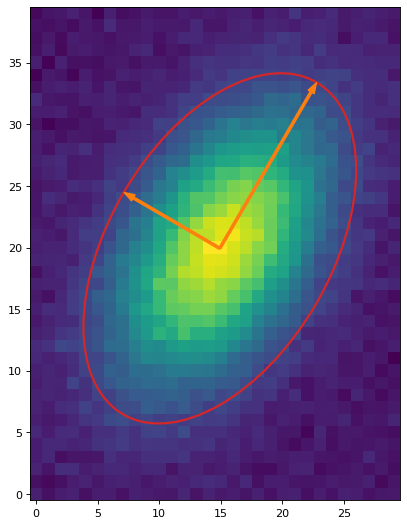# Morphological Properties (`photutils.morphology`)¶

## Introduction¶

The `data_properties()` function can be used to calculate the morphological properties of a single source in a cutout image. `data_properties` returns a `SourceCatalog` object. Please see `SourceCatalog` for the list of the many properties that are calculated. Even more properties are likely to be added in the future.

If you have a segmentation image, the `SourceCatalog` class can be used to calculate the properties for all (or a specified subset) of the segmented sources. Please see Source Photometry and Properties from Image Segmentation for more details.

## Getting Started¶

Let’s extract a single object from a synthetic dataset and find calculate its morphological properties. For this example, we will subtract the background using simple sigma-clipped statistics.

First, we create the source image and subtract its background:

```>>> from photutils.datasets import make_4gaussians_image
>>> from astropy.stats import sigma_clipped_stats
>>> data = make_4gaussians_image()[43:79, 76:104]
>>> mean, median, std = sigma_clipped_stats(data, sigma=3.0)
>>> data -= median  # subtract background
```

Then, calculate its properties:

```>>> from photutils.morphology import data_properties
>>> cat = data_properties(data)
>>> columns = ['label', 'xcentroid', 'ycentroid', 'semimajor_sigma',
...            'semiminor_sigma', 'orientation']
>>> tbl = cat.to_table(columns=columns)
>>> tbl['xcentroid'].info.format = '.10f'  # optional format
>>> tbl['ycentroid'].info.format = '.10f'
>>> tbl['semiminor_sigma'].info.format = '.10f'
>>> tbl['orientation'].info.format = '.10f'
>>> print(tbl)
label   xcentroid     ycentroid   ... semiminor_sigma  orientation
...       pix            deg
----- ------------- ------------- ... --------------- -------------
1 14.0225090502 16.9901801466 ...    3.6977761870 60.1283048753
```

Now let’s use the measured morphological properties to define an approximate isophotal ellipse for the source:

```>>> import astropy.units as u
>>> from photutils.aperture import EllipticalAperture
>>> position = (cat.xcentroid, cat.ycentroid)
>>> r = 3.0  # approximate isophotal extent
>>> a = cat.semimajor_sigma.value * r
>>> b = cat.semiminor_sigma.value * r
>>> apertures = EllipticalAperture(position, a, b, theta=theta)
>>> plt.imshow(data, origin='lower', cmap='viridis',
...            interpolation='nearest')
>>> apertures.plot(color='#d62728')
```## Reference/API¶

This subpackage contains tools for measuring morphological properties of objects in an astronomical image.

### Functions¶

 `data_properties`(data[, mask, background]) Calculate the morphological properties (and centroid) of a 2D array (e.g., an image cutout of an object) using image moments. `gini`(data) Calculate the Gini coefficient of a 2D array.